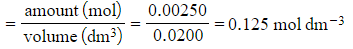# Edexcel A Level Chemistry:复习笔记1.7.2 Titrations

### Titration Calculations

#### Volumetric Analysis

• Volumetric analysis is a process that uses the volume and concentration of one chemical reactant (standard solution) to determine the concentration of another unknown solution
• The technique most commonly used is a titration
• The volumes are measured using two precise pieces of equipment, a volumetric or graduated pipette and a burette
• Before the titration can be done, the standard solution must be prepared
• Specific apparatus must be used both when preparing the standard solution and when completing the titration, to ensure that volumes are measured precisely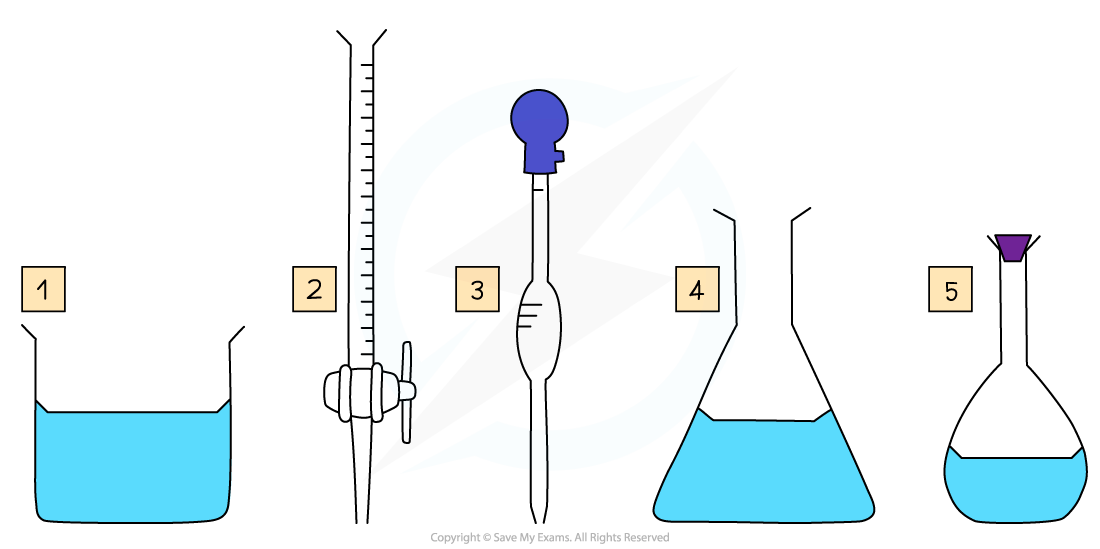Some key pieces of apparatus used to prepare a volumetric solution and perform a simple titration

1. Beaker
2. Burette
3. Volumetric Pipette

#### Making a Standard Solution

• Chemists routinely prepare solutions needed for analysis, whose concentrations are known precisely
• These solutions are termed volumetric solutions or standard solutions
• They are made as accurately and precisely as possible using three decimal place balances and volumetric flasks to reduce the impact of measurement uncertainties
• The steps are: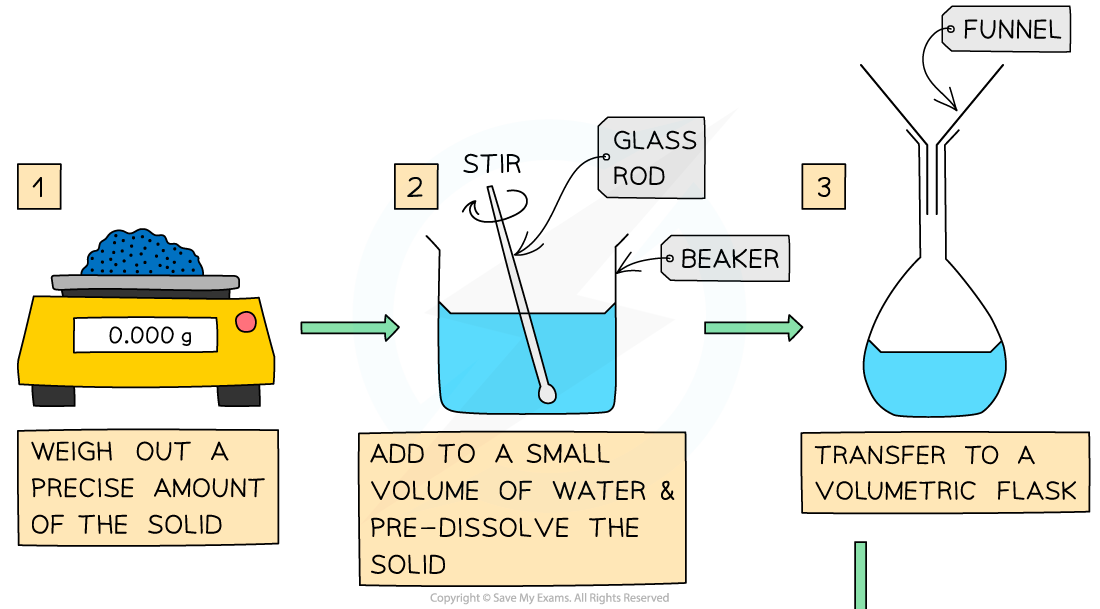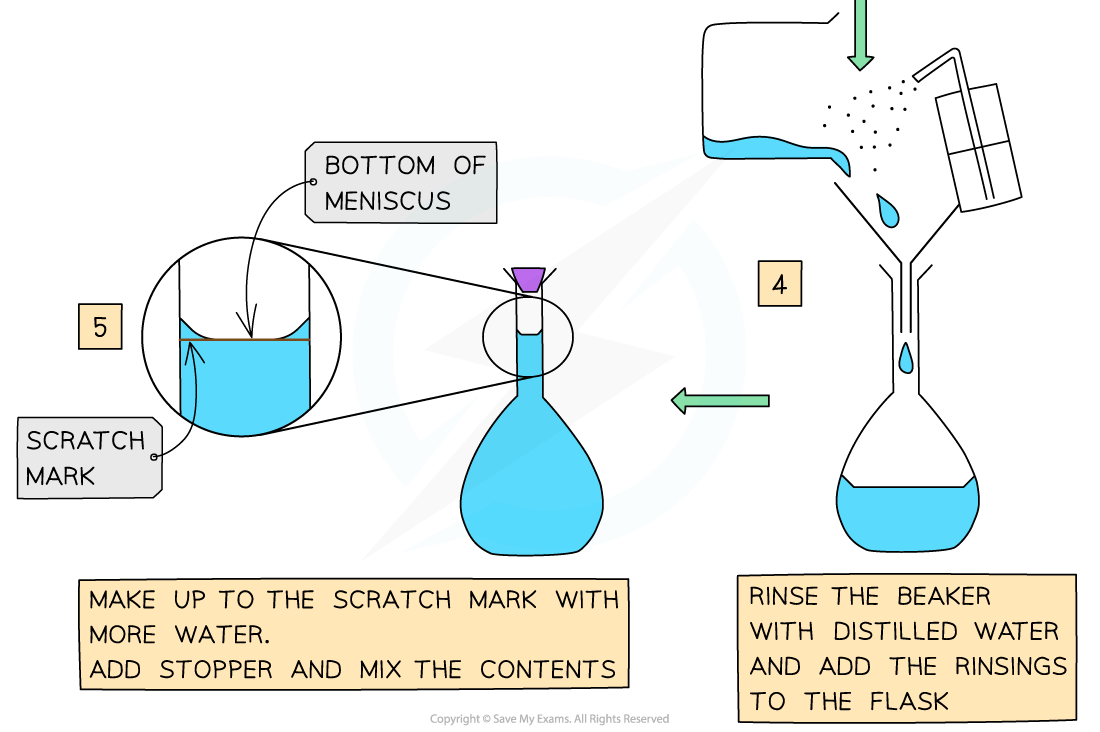#### Performing the Titration

• The key piece of equipment used in the titration is the burette
• Burettes are usually marked to a precision of 0.10 cm3
• Since they are analogue instruments, the uncertainty is recorded to half the smallest marking, in other words to ±0.05 cm3

• The end point or equivalence point occurs when the two solutions have reacted completely and is shown with the use of an indicator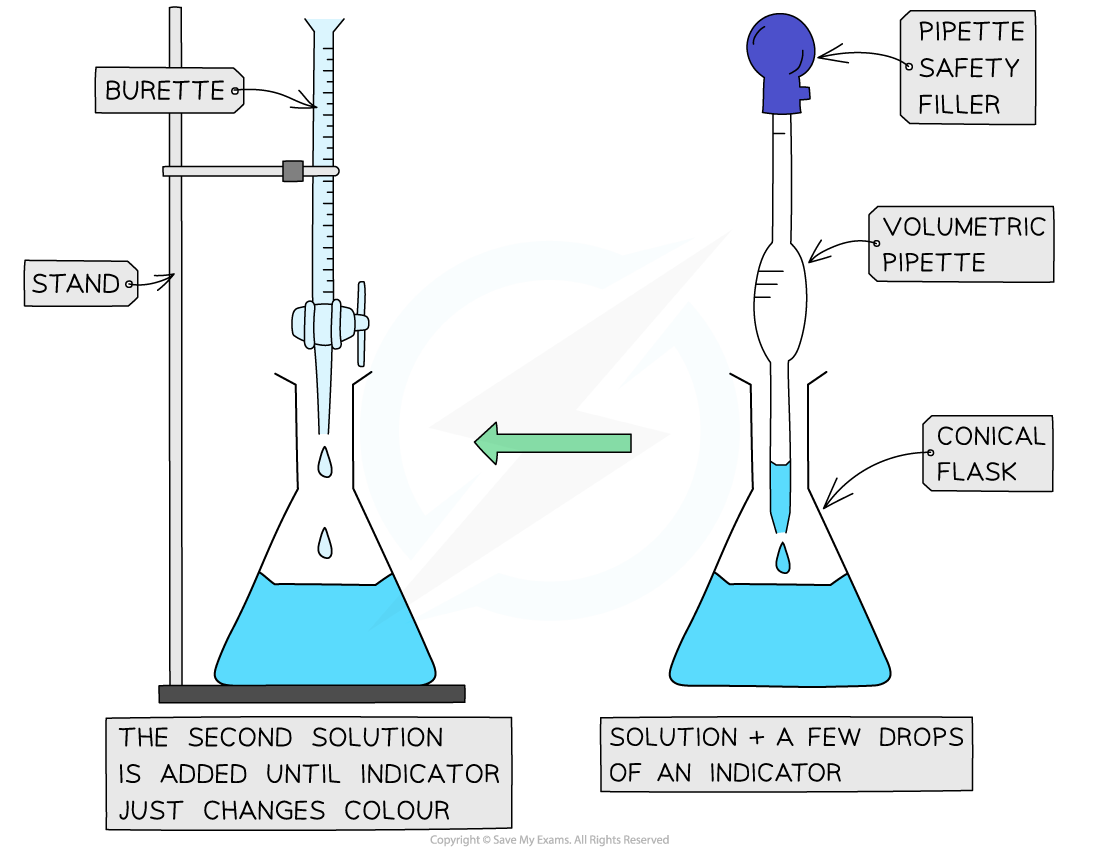The steps in a titration

• A white tile is placed under the conical flask while the titration is performed, to make it easier to see the colour change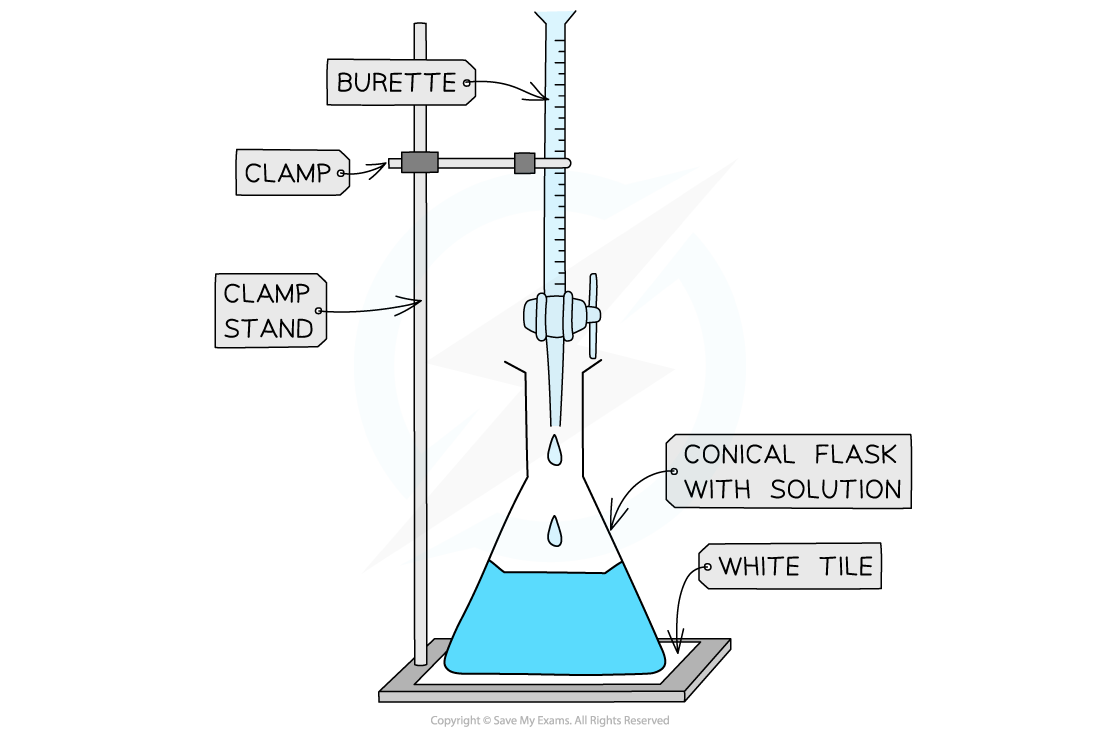The steps in a titration

• The steps in a titration are:
• Measuring a known volume (usually 20 or 25 cm3) of one of the solutions with a volumetric pipette and placing it into a conical flask
• The other solution is placed in the burette
• To start with, the burette will usually be filled to 0.00 cm3

• A few drops of the indicator are added to the solution in the conical flask
• The tap on the burette is carefully opened and the solution added, portion by portion, to the conical flask until the indicator starts to change colour
• As you start getting near to the end point, the flow of the burette should be slowed right down so that the solution is added dropwise
• You should be able to close the tap on the burette after one drop has caused the colour change

• Multiple runs are carried out until concordant results are obtained
• Concordant results are within 0.1 cm3 of each other

#### Recording and processing titration results

• Both the initial and final burette readings should be recorded and shown to a precision of  ±0.05 cm3, the same as the uncertaintyA typical layout and set of titration results

• The volume delivered (titre) is calculated and recorded to an uncertainty of ±0.10 cm3
• The uncertainty is doubled, because two burette readings are made to obtain the titre (V final – V initial), following the rules for propagation of uncertainties

• Concordant results are then averaged, and non-concordant results are discarded
• The appropriate calculations are then done

#### Volumes & concentrations of solutions

• The concentration of a solution is the amount of solute dissolved in a solvent to make 1 dm3 of  solution
• The solute is the substance that dissolves in a solvent to form a solution
• The solvent is often water

Concentration (mol dm-3) =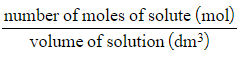• A concentrated solution is a solution that has a high concentration of solute
• A dilute solution is a solution with a low concentration of solute
• When carrying out calculations involve concentrations in mol dm-3 the following points need to be considered:
• Change mass in grams to moles
• Change cm3 to dm3

• To calculate the mass of a substance present in solution of known concentration and volume:
• Rearrange the concentration equation

number of moles (mol) = concentration (mol dm-3) x volume (dm3)

• Multiply the moles of solute by its molar mass

mass of solute (g) = number of moles (mol) x molar mass (g mol-1)

#### Worked Example

Neutralisation calculation
25.0 cm3 of 0.050 mol dm-3 sodium carbonate was completely neutralised by 20.00 cm3  of dilute hydrochloric acid. Calculate the concentration in mol dm-3 of hydrochloric acid.

Step 1: Write the balanced symbol equation

Na2CO3  +  2HCl  →  2NaCl  +  H2O  +  CO2

Step 2: Calculate the amount, in moles, of sodium carbonate reacted by rearranging the equation for amount of substance (mol) and dividing the volume by 1000 to convert cm3 to dm3

• Amount (Na2CO3) = 0.025 dm3 x 0.050 mol dm-3 = 0.00125 mol

Step 3: Calculate the moles of hydrochloric acid required using the reaction’s stoichiometry

• 1 mol of Na2CO3 reacts with 2 mol of HCl, so the molar ratio is 1 : 2
• Therefore 0.00125 moles of Na2CO3 react with 0.00250 moles of HCl

Step 4: Calculate the concentration, in mol dm-3, of hydrochloric acid

• [HCl]